# Tesla Capital Structure and Debt Leverage Is Changing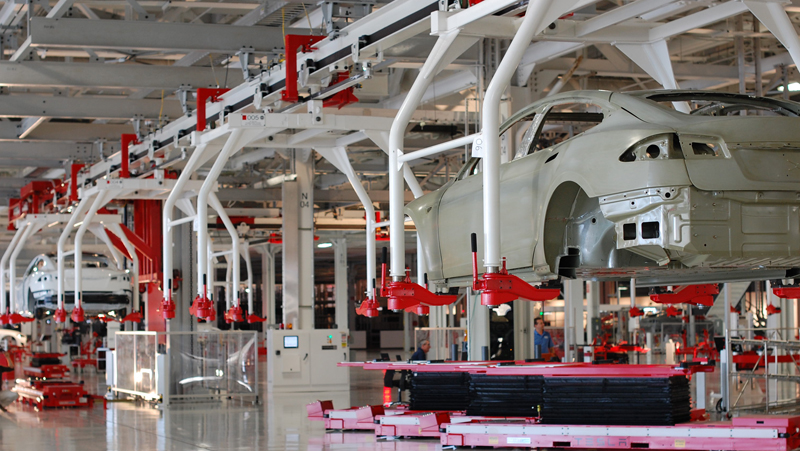A Tesla factory in Fremont, CA. Source: Flickr

Tesla (NASDAQ: TSLA) debt to equity ratio is a metric that measure the debt leverage with respect to equity of the company.

The equity is the net worth or book value of Tesla.

The more debt Tesla has, the higher the debt to equity ratio is.

For example, if Tesla has \$1 dollars of equity and \$10 dollars of debt, its debt to equity ratio will be 10 to 1 or 10X, meaning that Tesla is leveraging 10X higher than its net worth.

In fact, Tesla is highly leveraged in this case.

Other than debt leverage, from the debt to equity ratio, we can also find out about the capital structure of Tesla.

From the ratio, we can find out how Tesla funded its balance sheets, whether entirely by debts or by equity or equally both.

Ideally, the capital structure of a company should be well balanced between debts and equity.

For example, for every 1 dollar of equity, there is 1 dollar of debt. In this case, the debt to equity ratio will be 1.

However, in real life, this balanced debt structure scenario is not always the case for most companies.

The reason is that it may not be prudent to rely largely on equity finances.

In most cases, the cost of debt may be much cheaper than the required rate of return on equity.

This is particularly true in a low-interest-rate environment.

In this article, we will explore Tesla’s capital structure to find out how the company leverages using debts and equity.

Let’s get started!

## Equation for Debt to Equity Ratio

Before illustrating further, the equation that I used to calculate the debt to equity ratio is shown as below:

Debt to Equity Ratio = Debt / Equity

From the above equation, the equity part is easily understood.

Basically, it’s the total equity or the book value that can be easily obtained from the balance sheets.

## Tesla’s Equity

Tesla’s equities include both shareholders’ equity and minority interest equity.

Two types of equities exist because Tesla is a holding company of multiple subsidiaries.

As such, the balance sheet is consolidated with the assets and liabilities of all subsidiaries.

The consolidation has led to the existence of minority interests in the balance sheet since Tesla does not own 100% of some subsidiaries.

The following snapshot illustrates Tesla’s non-controlling interest or minority interest portion in the balance sheet.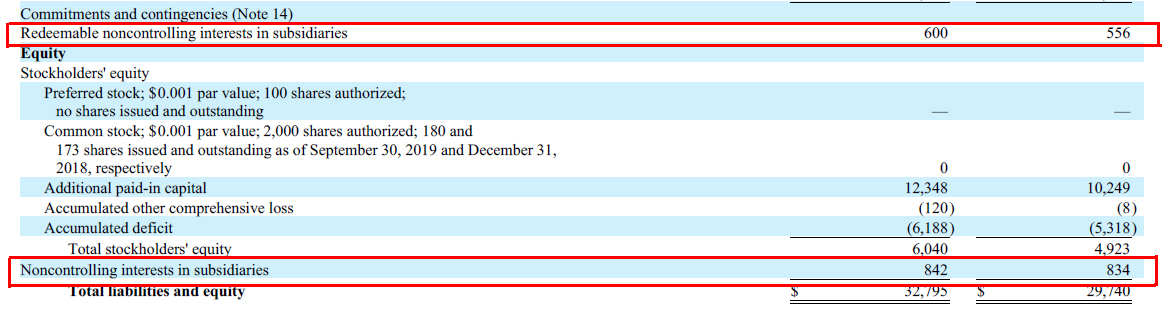Tesla minority interest

Tesla’s minority interest exists as shown in the balance sheet above is due mainly to the company’s ownership structure.

## Tesla’s Debt Definitions

From the debt to equity ratio, difficulties may arise when we try to figure out the debt part of the equation.

There are countless definitions of “debts”. In this case, debts can be derived from long-term or current debts, total liabilities or interest-bearing only debts.

For simplicity, I have considered only “debts” that matter most in the analysis of Tesla’s leverages.

The following shows the definition of debt in Tesla’s case:

1. Total debts – debt instruments that include both long and short-term loans as well as finance and operating leases. Basically, total debts are all interest-bearing debt instruments.

2. Long-term liabilities – these are long-term loans and other miscellaneous liabilities such as accounts payable, deferred revenues, leases, warranty reserves and so on. Only the long-term portion is measured in this case.

3. Total liabilities – these are all the liabilities in the balance sheet that are comprised of both long and short-term loans as well as other long and short liabilities such as accounts payable, deferred revenues and other miscellaneous liabilities.

The subsequent debt to equity ratio discussion will revolve around these 3 types of “debt” definitions mentioned above.

## Tesla’s Total DebtTesla debt and leases

* Total debt figures come from the author’s own calculation and consists of long and short-term debt instruments as well as leases.

Let’s first briefly look at Tesla’s total debt as shown in the chart above.

As of 2021 Q1, Tesla’s total debt reached nearly \$13 billion, which was 18% lower compared to the same quarter a year ago.

As briefly mentioned in the prior discussion, Tesla’s total debt consists of mainly interest-bearing debt instruments, including long-term and current loans.

Additionally, Tesla’s total debt also includes both finance and operating leases.

For your information, finance and operating leases are interest-bearing debt instruments.

In fact, they have added substantial indebtedness to the company as shown in this article: Tesla debts analysis.

The leases, both operating and finance, are the total minimum lease payments or MLPs which also include the interest portion.

The following snapshot shows an example of Tesla’s minimum lease payments disclosed in one of its financial statements: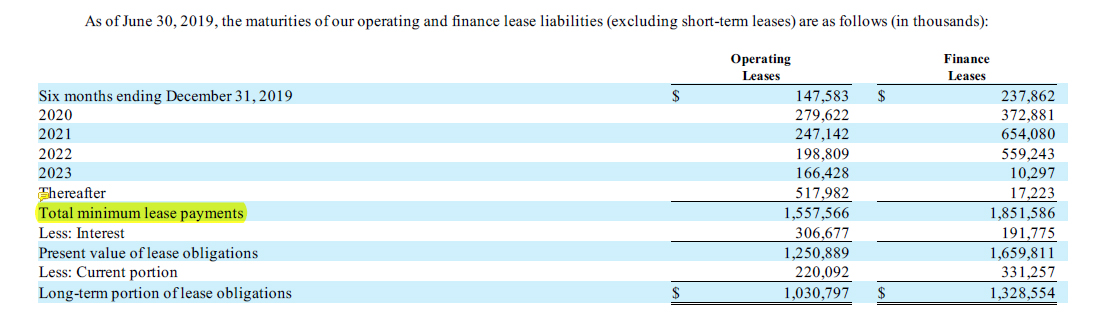Tesla operating and finance leases from the quarterly statement

Therefore, Tesla’s total debt does not include such liabilities as accounts payable, accrued liabilities, deferred revenue, warranty reserves and so on.

In short, Tesla’s total debt consists of only interest-bearing debt instruments and leases, both finance and operating.

## Tesla’s Total Debts to Equity RatioTesla debt to equity ratio

* Debt to equity ratio is calculated by the author using Tesla’s total debt and equity obtained from the company’s balance sheets.
* Total debt consists of long and short-term debt as well as leases.

The chart above shows Tesla’s total debts to equity ratio for the period from 2015 to 2021.

As seen from the chart, Tesla’s total debt to equity ratio was the highest back in 2015 when the ratio averaged around 4.0X.

At this ratio, Tesla’s leverage was \$4 dollars of debt to \$1 dollars of equity, illustrating that the company was moderately leveraged with respect to equity.

Over the years, Tesla’s debt to equity ratio has been declining and reached only 0.5X as of 2021 1Q.

At this ratio, Tesla’s leverage was low as its debt was less than its equity.

There are a few reasons for Tesla’s declining debt leverage.

First, Tesla has paid off some of its outstanding debt.

Also, Tesla has taken on fewer borrowings to fund its expansion in recent years and thus, the smaller debt to equity ratio.

Secondly, Tesla has issued more equity in recent years to fund its expansion.

This approach makes sense as Tesla’s share price has been surging to new highs.

In this case, Tesla can issue less equity to obtain the same amount of capital.

Also, equity is less risky compared to debt because Tesla does not have to repay the capital obtained through equity.

The only downside to equity is that it dilutes the control of the company.

For debt, Tesla needs to pay back not only the principal but also the interest portion.

## Tesla Long-Term Liabilities to Equity Ratio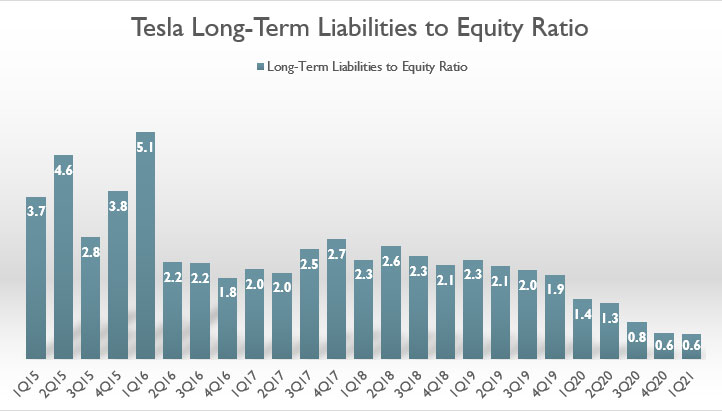Tesla long-term liabilities to equity ratio

* Long-term liabilities to equity ratio is calculated by the author using Tesla’s long-term liabilities and equity obtained from the company’s balance sheets.
* Long-term liabilities consists of all long-term debt and other liabilities such as pension funds, taxes due, etc.

In terms of long-term liabilities, Tesla’s long-term liabilities to equity ratio plot looks very similar to the total debts to equity ratio plot.

In fact, the trend of the long-term liabilities to equity ratio plot looks exactly the same as the prior chart.

For instance, Tesla was highly leveraged back in 2015 when the long-term liabilities to equity ratio was averaged around 3.8X.

Over the years, the ratio has significantly declined and reached only 0.6X as of Q1 2021.

At this ratio, Tesla was minimally leveraged at \$0.60 dollar of long-term liabilities to \$1.00 dollar of equity.

Similarly, Tesla’s declining total liabilities to equity ratio can be attributed to the declining debt and decreasing indebtedness of the company in recent years.

Additionally, Tesla’s surging stock price has caused equity issuance to be less risky and cheaper compared to debt borrowings.

In this case, Tesla can issue fewer equities to obtain a larger capital.

## Tesla Total Liabilities to Equity Ratio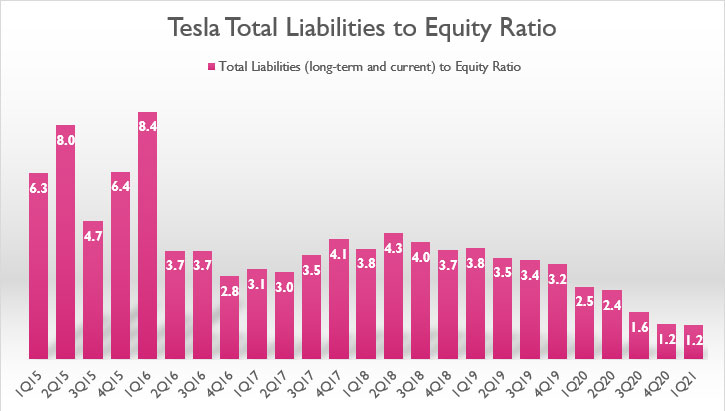Tesla total liabilities to equity ratio

* Total liabilities to equity ratio is calculated by the author using Tesla’s total liabilities and equity obtained from the company’s balance sheets.
* Total liabilities consists of all long-term and current debt and other liabilities such as accounts payable, accrued liabilities, pension funds, taxes due, etc.

The chart above shows Tesla’s total liabilities to equity ratio for the past 6 years from 2015 to 2021.

Tesla’s total liabilities are basically all long and short-term borrowings and whatever the company owes to 3rd parties in the balance sheets.

In terms of total liabilities, the trend of Tesla’s total liabilities to equity ratio also looks similar to prior charts.

The only difference is that the leverage ratio has been much higher in the current chart.

This is expected as all liabilities are included in the measurement of the debt to equity ratio.

Again, a similar trend is observed for Tesla’s total liabilities to equity ratio.

In this case, Tesla used to have a higher ratio at more than 4.0X in 2015.

However, this ratio has since declined and reached only 1.2X in 1Q 2021, the lowest the company has ever seen.

At this ratio, Tesla is minimally leveraged at \$1.20 dollar of liabilities to \$1.00 dollar of equity.

Tesla has been getting rid of debt and has inclined to take on more debt in recent quarters.

At the same time, Tesla’s other liabilities have remained flat.

Additionally, Tesla’s valuation has been rising steadily and has surged significantly in recent quarters.

Therefore, it is to Tesla’s advantage to obtain capital through equity issuance since the company can issue less equity but is able to get a much larger capital.

## Tesla’s Capital Structure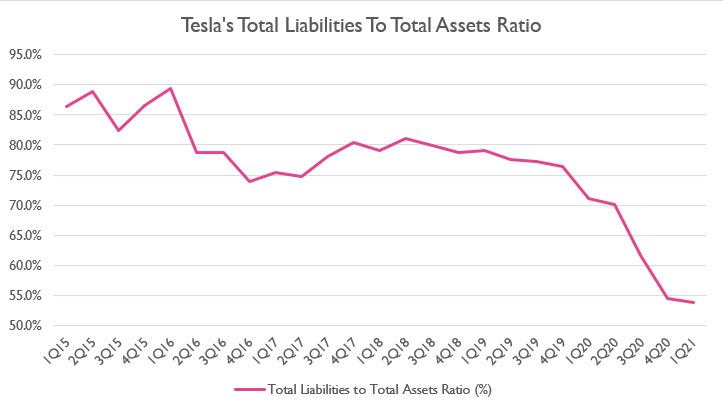Tesla total liabilities to asset ratio

* Total liabilities to asset ratio is calculated by the author using Tesla’s total liabilities and total assets obtained from the company’s balance sheets.

The equation to calculate the total liabilities to total asset ratio is shown below:

Total Liabilities to Asset Ratio = [ Total Liabilities / Total Assets ] X 100%

Tesla’s capital structure can be obtained from the total liabilities to total assets ratio.

As shown in the chart above, Tesla’s capital structure was mostly debt or liabilities prior to 2020.

For example, Tesla’s total liabilities to total asset ratio had been above 75% between 2015 and 2019, indicating that the company had been entirely funded by liabilities.

However, the ratio has significantly declined in recent quarters and reached only 55% in 2021 Q1, a record low for Tesla.

At this ratio, Tesla’s capital structure was 55% of liabilities and 45% of equity.

Tesla’s total liabilities to asset ratio dropped notably fast in 2020.

In this case, Tesla’s equity issuance has significantly soared in 2020, thereby leading to the declining ratio.

This scenario is expected as Tesla’s stock price has been surging to multiple highs lately, with the company’s market cap reaching nearly \$800 billion at one point.

While Tesla has relied less on debt to fund its expansion, its capital structure was still pretty much made up of liabilities at this ratio.

Nevertheless, Tesla is using more equity to fund its expansion now.

At this composition, Tesla’s debt leverage was moderately low at about \$0.55 dollars of liabilities to \$1.00 dollars of assets in Q1 2021.

## Tesla’s Debt to Asset Ratio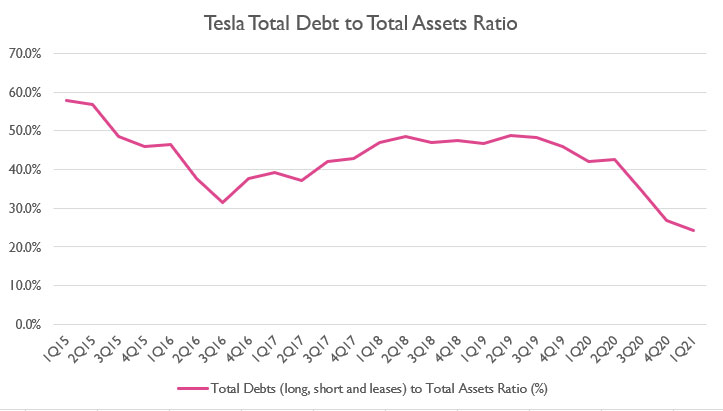Tesla total debt to asset ratio

* Total debt to asset ratio is calculated by the author using Tesla’s total debt and total assets obtained from the company’s balance sheets.

We can look at Tesla’s debt instead of its total liabilities with respect to total assets.

In this case, the debt to asset ratio is what we need to look at Tesla’s debt leverage with respect to assets.

As shown in the chart above, Tesla used to have a debt to asset ratio as high as 60% in 2015.

However, this figure has since declined and reached only 24% as of Q1 2021.

At this ratio, Tesla’s capital structure was 24% debt and the rest was equity as well as other liabilities.

Again, Tesla was minimally leveraged at this ratio and has \$0.24 dollar of debt to \$1.00 dollar of assets.

The downtrend observed in Tesla’s debt to asset ratio reinforces the idea that Tesla has been slowly shifting away from debt to fund its expansion.

Instead, Tesla uses more equity as its main source of funding.

## Conclusion

To recap, Tesla’s total debts to equity ratio has significantly declined from its peak value of more than 4.0 in 2015 to only 0.5 as of 2021 Q1.

The downtrend is also observed for the total liabilities to equity ratio, indicating that Tesla’s preference for funding has shifted towards equity over debt.

The trend is expected when Tesla’s valuation is slowly gaining ground and has surpassed the \$600 billion level by 1Q 2021.

At this enormous valuation, Tesla can obtain a higher capital through equity issuance even though this practice may mean further dilution to its existing stockholders.

## References and Credits

1. Financial figures in all charts above were obtained and referenced from Tesla’s annual and quarterly statements available in Tesla Quarterly Results.

2. Featured image was used under Creative Common Licenses and obtained from mangopulp2008 and Maurizio Pesce.

## Disclosure

Readers, investors, analysts, bloggers, visitors, researchers, writers, or academicians are highly encouraged to use, copy, quote, distribute, duplicate, modify, edit, upload, download, share and link any materials on this webpage such as the charts, snapshots, texts, paragraphs, etc. in your websites, research papers, essays and so on.

You can credit back to this page by a link or a mention of the website. Thanks for sharing!

•Anna December 18, 2020, 7:21 am
•cckean December 18, 2020, 9:42 am